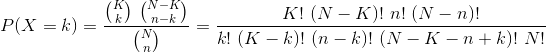# Use Prime Factorization for Computations

Use Prime Factorization for simplifying computations, for instance for ratios of large factorials.

R package that uses Prime Factorization for computations.

This small R package was initially developed to compute hypergeometric probabilities which are used in Fisher's exact test, for instance.

## Features

### Main feature

To computeyou can use `ComputeDivFact(c(K, (N-K), n, (N-n)), c(k, (K-k), (n-k), (N-K-n+k), N))`. This uses a Prime Factorization to simplify computations.

### Play with primes

You can also test if a number is a prime and get all prime numbers up to a certain number.

# Reference manual

install.packages("primefactr")

0.1.1 by Florian Privé, 3 years ago

https://github.com/privefl/primefactr

Report a bug at https://github.com/privefl/primefactr/issues

Browse source code at https://github.com/cran/primefactr

Authors: Florian Privé [aut, cre]

Documentation:   PDF Manual